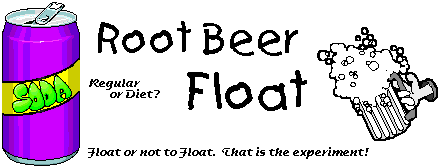What is needed

• One can of root beer, and one can of diet root beer
• A large container of water. A deep sink or a bathtub full of water will work fine.

What to do

Take one of the cans in your left hand and one of the cans in your right. They probably feel about the same; same size, same weight. Now set both of the cans in your large container of water. What happens? One of them sinks and one of them floats.

What is happening

Do you know why this happens? Do you know why ANYTHING floats in water rather than sinks. The reason has to do with relative densities of materials. The density of water is 1g/cm^3. If the density of an object is less than one, then the object floats in water. If the density of an object is more than one, the object will sink in water.

Now we know that the density of one of the cans is greater than 1 g/cm^3 and the density of the other is less than 1 g/cm^3. What could account for the difference? Let's assume that there is the same amount of liquid and air inside each of the cans. Let's also assume that there is the same amount of aluminum in each of the cans. NOW what can you think of that could make the different in densities.

The answer is that there is a difference in density between the two cans because of the difference in density between sugar and the sugar substitute used in diet soft drinks.

 The contents of the page was modified from that created for the Little Shop of Physics, Colorado State University

Follow-up

1. What is the density of water?

2. We know that onjects that are observed to float in water must have what density?

3. If the density of an object is greater than 1 g/cm^3, what will happen if it is placed in water?

4. What happened when you placed the root beer and the diet root beer into the water?

5. What explains the difference in the densities of the two sodas?

6. Which soda has a density greater than 1 g/cm^3? Which had a density less than 1 g/cm^3?

 Obtaining credit for your efforts Print a copy of the blank laboratory write-up paper. Read and complete each section, incomplete write-ups receive no credit. Have your parent check your work and sign the paper.